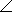# Electrical Engineering - RLC Circuits and Resonance - Discussion

Discussion Forum : RLC Circuits and Resonance - General Questions (Q.No. 21)
21.
A certain series RLC circuit with a 200 Hz, 15 V ac source has the following values: R = 12, C = 80F, and L = 10 mH. The total impedance, expressed in polar form, is
12.2812.34°12.5712.34°9.9512.34°12.6212.34°Explanation:
No answer description is available. Let's discuss.
Discussion:
6 comments Page 1 of 1.

SANJAY said:   9 years ago
Z = R+j(Xl-Xc).

Xl = 2*3.14*f*l.

Xc = 1/(2*3.14*f*c).

Option A.

Arun said:   8 years ago
How is this calculation please explain?

GAYATRI said:   8 years ago
Z = sq.root of [r2+(xl-xc)2] where,

Xl = 2*3.14*f*l = 2*3.14*200*10x10-3 = 12.56637.

Xc = 1/(2*3.14*f*c) = 1/(2*3.14*200*80*10-6) = 9.947.

Z = Sq.root of [12*12+(12.566 - 9.947)*(12.566 - 9.947)].

= Sq.root of [144+(2.619*2.619).

= Sq.root of [144+6.86].

= Sq.root of 150.860.

= 12.28.

Quest77 said:   5 years ago
The angle should be 29.99 degrees. Inverse tangent of (xl-xc)\r.

HAris said:   3 years ago
Z=√R^2+(XL-XC)^2
Z=√12^2+((2*PIE*F*L)-(1/2*PIE*F*C))^2
Z=√144+12.566-9.947.

Z=12.28 ohm.

FOR ANGLE θ due to Polar Form as Required,
θ = tangent inverse*(XL-XC/R).
= tan.inv*(12.566-9.947/12).
= 12.31.
So,
= 12.28<12.31* ohm , Option A.

SONAL said:   1 year ago
@Haris.

How to calculate the below step? Please explain.

Î¸ = tangent inverse*(XL-XC/R).
= tan.inv*(12.566-9.947/12).
= 12.31.